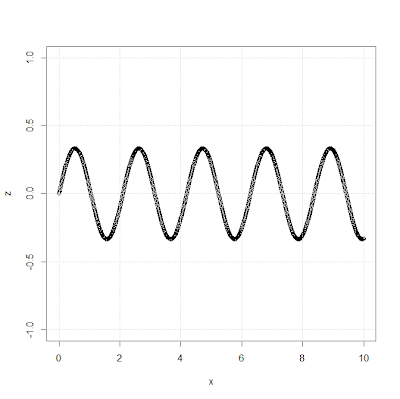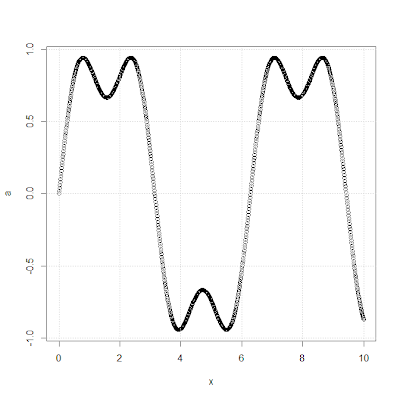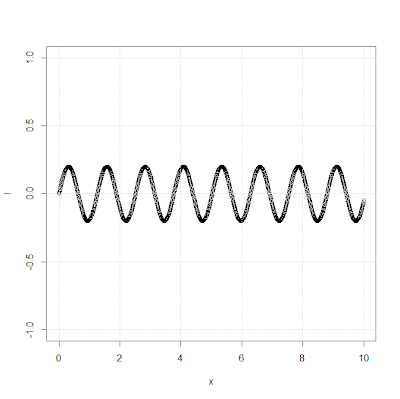Abstract New

Fourier analysis - the Fourier series and Fourier transform explained without formulae

At first there was Fourier

Joseph Fourier, a french mathematician embarked upon a quest to derive an equation that could describe the distribution of heat in solid bodies. Fourier built on existing theories that tried to breakdown a periodic function into a series of oscillating functions. He discovered that any periodic signal can be represented by a superposition of sines and cosines. Mathematically it meant - given any function one could easily break it up into a bunch of simpler basis functions. Every periodic signal can then be constructed (replicated) by a combination of these basic building blocks.

Fourier Series

Consider a square wave with a period of T. The fundamental frequency of the wave is f (= 1/T). The Fourier series states that this signal can be approximated by a sum of sine and cosine waves with frequencies that are integral multiples of the fundamental frequency.

To understand this better let us try to approximate the square wave (Shown in Fig 1) using the sum of sine waves with frequencies that are multiples of the fundamental frequency (f). As we include higher orders of frequencies (Figures 2,3, and 5), the sum of these individual sine waves begins to approximate the square wave better. Note that the amplitudes of each wave is different. The Fourier Analysis helps derive the amplitudes of each of these sine waves. Figure 6 shows the sum of the first three orders of the sine waves and you will begin to see that it start to approximate the square wave. The higher the orders, the better the approximation.Fig. 1 - Sample Square Wave with a frequency = fFig. 2 - First order sine wave with a frequency = fFig. 3 - Sine wave of the second order. Frequency = 2*fFig. 4 - Sum of the first two orders of sine wavesFig. 5 - Sine wave of the third order Frequency = 3*fFig. 6 - Sum of the first three orders of sine waves

Fourier Series vs Fourier Transform

At this point, the inquisitive reader is wondering about what happens to aperiodic signals. Enter the Fourier transform. The Fourier transform is a generalization of the Fourier series that applies to all signals. All signals can be decomposed into their constituent basis functions (in this case the sinusoids) by extending the intervals to infinity.

As the signal’s time period tends to infinity the fundamental frequency tends to 0.

Instead of taking the building blocks whose frequencies are multiples of the fundamental frequency, (i.e. those that would fit within the time period of the periodic signal) we now need to consider all possible frequencies.

For a more detailed explanation of the Fourier transform take a look at this article

Real world applications of Fourier Analysis

Filtering

Fourier transform is widely used to filter signals. For example, to filter high frequency noise out of an audio signal, perform a Fourier transform on the signal and set the amplitude of the high frequency components to zero. The inverse Fourier transform is then used to convert the filtered signal back to the time domain. In image processing, high frequencies often need to be eliminated to make an image smoother. The image is converted to the frequency domain using the Fourier transform. The high frequency components are attenuated and the result is converted back to a smoother image.

Noise cancelling headsets use the Fourier transform to cancel out external noise. The headsets have an microphone that captures the external noise. It then extracts the constituent frequencies by using Fourier analysis and emits phase shifted waves at corresponding frequencies. The two signals cancel out each other and the user does not hear the external noise.

Signal compression

The inverse Fourier transform can be used to recreate a signal from a set of frequencies and their amplitudes. The Fourier transform can be used to enable lossy compression of signals by reducing the number of Fourier components used to represent the signal.